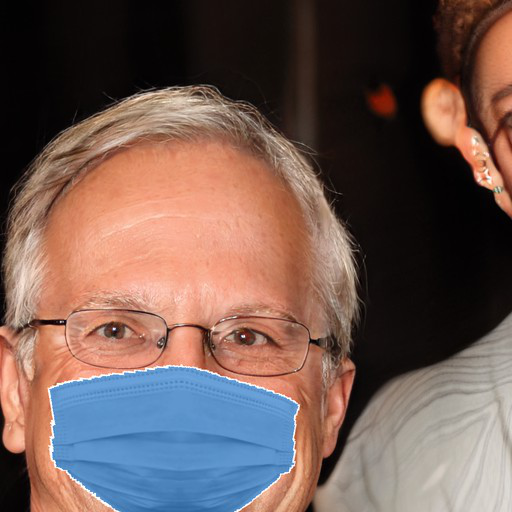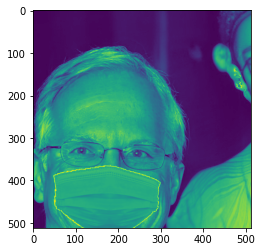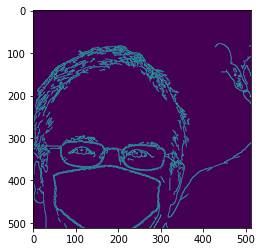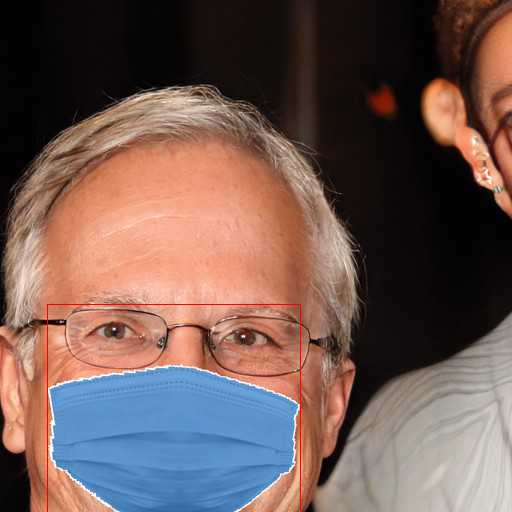# [ Baseline ] Mask Prediction

The baseline with a naive approach of mask detection using Canny Edge Detection, Sklearn Models etc.## Getting Started with Mask Recognition Challenge

In this puzzle, we have detect mask type and bounding box of the mask from the image of human face.

In this notebook, we'll use the naive approach to prepare the baseline for this puzzle.

## Naive Approach¶

• We will create bounding box that will detect the face and crop the image as per the co-ordinates of bounding box from image.
• Once we've the cropped image(It'll have the image of mask), we'll feed the data to train classifier which will classify the mask.

# Setting up Environment¶

So we will first need to download the python library by AIcrowd that will allow us to download the dataset by just inputting the API key.

In [ ]:
%%capture
!pip install aicrowd-cli



In [ ]:
%aicrowd login

Please login here: https://api.aicrowd.com/auth/24dM8PQYqbc2pXF9DN3V-k7To624IOvhM1jLreSiZxw
API Key valid
Saved details successfully!


We will create a folder name data and download the files there.

In [ ]:
!rm -rf data
!mkdir data
%aicrowd ds dl -c mask-prediction -o data

In [ ]:
!unzip data/train.zip -d data/ > /dev/null
!unzip data/val.zip -d data/ > /dev/null
!unzip data/test.zip -d data/ > /dev/null


# Importing Libraries¶

In [ ]:
#Reading the file
import pandas as pd
import numpy as np
import os

from PIL import Image, ImageDraw
import cv2
import matplotlib.pyplot as plt
import numpy as np

# Misc.
from tqdm.notebook import tqdm
from sklearn.ensemble import RandomForestClassifier


# Diving in the dataset 🕵️‍♂️¶

In [ ]:
train_images = 'data/train'
val_images = 'data/val'
test_images = 'data/test'

In [ ]:
train_df = pd.read_csv("data/train.csv")

In [ ]:
train_df.head()

Out[ ]:
0 k8o0f [73, 197, 293, 400] N95
1 7a0l9 [47, 364, 300, 512] surgical
2 wfp7p [203, 221, 380, 403] N95
3 7qaw6 [87, 210, 322, 385] surgical
4 i4kqj [227, 283, 479, 475] surgical
In [ ]:
train_df.loc[train_df.masktype == "cloth"][:2]

Out[ ]:
5 uokc1 [3, 240, 276, 441] cloth
13 qzbds [91, 321, 380, 512] cloth
In [ ]:
train_img = os.listdir(train_images)
train_img[:5]

Out[ ]:
['urko1.jpg', 'ngb1h.jpg', 'u54pu.jpg', 'wvkoh.jpg', 'ou7fd.jpg']

From the above, we can see that ImageId is image_name without extensions(.jpg).

In [ ]:
img = Image.open(os.path.join(train_images, '7a0l9.jpg'))
img

Out[ ]:# Image Preprocessing¶

In this section we are going to learn some opencv functions which can help us detecting the mask from the image!

In [ ]:
# Converting the image to numpy array
np_img= np.array(img)
np_img.shape

Out[ ]:
(512, 512, 3)
In [ ]:
# Converting the Image to RGB to Grayscale ( black/white )
gray= cv2.cvtColor(np_img, cv2.COLOR_BGR2GRAY)
plt.imshow(gray)

Out[ ]:
<matplotlib.image.AxesImage at 0x7fed5dd8b850>### cv2.Canny¶

Now cv2.Canny is Canny Edge Detection which helps us to detect edges in the image. Let's try it our on the image

In [ ]:
canny= cv2.Canny(gray, 120,250)
plt.imshow(canny)

Out[ ]:
<matplotlib.image.AxesImage at 0x7fed5b845310>So as you can see the function detected edges including the mask and some noise too, there are many ways to reduce that noise, however you can try changing the parameters in the function and see where that leads.

### Countours¶

Contours are lines joining along the bounding of a intensity or color in an image. In the canny image or the original image, we see that the image has much different color as compared to the sky.

In [ ]:
# Finding contours in the image
contours, hierarchy = cv2.findContours(canny,
cv2.RETR_EXTERNAL, cv2.CHAIN_APPROX_NONE)

In [ ]:
# Sorting the contours in ascending order
contours = sorted(contours, key=cv2.contourArea)

In [ ]:
# Getting the bounding boxes of the biggest contours
x,y,w,h = list(cv2.boundingRect(contours[-1]))
x,y,w,h

Out[ ]:
(47, 304, 253, 208)
In [ ]:
# Showing the contour
draw_img = img.copy()
draw = ImageDraw.Draw(draw_img)
draw.rectangle([x,y,x+w,y+h ], outline ="red")
draw_img

Out[ ]:So as you can see, fnding contours did a pretty great job in finfing the mask.

In [ ]:
left,top,right, bottom = x,y,x+w,y+h

In [ ]:
mask = img.crop((left,top,right, bottom))